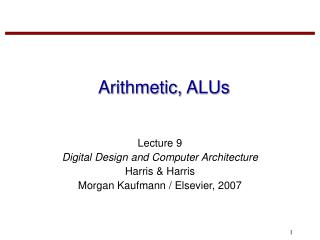# Arithmetic, ALUs - PowerPoint PPT PresentationDownload PresentationArithmetic, ALUs

Loading in 2 Seconds...

Arithmetic, ALUsDownload Presentation## Arithmetic, ALUs

- - - - - - - - - - - - - - - - - - - - - - - - - - - E N D - - - - - - - - - - - - - - - - - - - - - - - - - - -
##### Presentation Transcript

1. Arithmetic, ALUs Lecture 9 Digital Design and Computer Architecture Harris & Harris Morgan Kaufmann / Elsevier, 2007

2. 1-Bit Adders

3. Multi-bit Adder

4. Ripple-carry Adder

5. Subtractor

6. Comparator: Equality

7. Comparator: Less Than

8. Arithmetic Logic Unit (ALU)

9. ALU Design

10. ALU Function

11. Set Less Than (SLT)

12. Shifters • Logical shifter: shifts value to left or right and fills empty spaces with 0’s • Arithmetic shifter: same as logical shifter, but on right shift, fills empty spaces with the old most significant bit (msb). • Rotator: rotates bits in a circle, such that bits shifted off one end are shifted into the other end

13. Shifter Design

14. Example Write verilog code to implement the following function in hardware: y = b’c’ + ab’ Name the module “sillyfunction”.

15. Testbenches • Verilog code written to test other verilog modules • Not synthesizeable

16. Simple Testbench module testbench1(); reg a, b, c; wire y; // instantiate device under test sillyfunction dut(a, b, c, y); // apply inputs one at a time initial begin a = 0; b = 0; c = 0; #10; c = 1; #10; b = 1; c = 0; #10; c = 1; #10; a = 1; b = 0; c = 0; #10; c = 1; #10; b = 1; c = 0; #10; c = 1; #10; end endmodule

17. Self-checking Testbench module testbench2(); reg a, b, c; wire y; // instantiate device under test sillyfunction dut(a, b, c, y); // apply inputs one at a time // checking results initial begin a = 0; b = 0; c = 0; #10; if (y !== 1) \$display("000 failed."); c = 1; #10; if (y !== 0) \$display("001 failed."); b = 1; c = 0; #10; if (y !== 0) \$display("010 failed."); c = 1; #10; if (y !== 0) \$display("011 failed.");

18. Self-checking Testbench (cont.) a = 1; b = 0; c = 0; #10; if (y !== 1) \$display("100 failed."); c = 1; #10; if (y !== 1) \$display("101 failed."); b = 1; c = 0; #10; if (y !== 0) \$display("110 failed."); c = 1; #10; if (y !== 0) \$display("111 failed."); end endmodule

19. Testbench with Testvectors • Write testvectors file • Testbench: • Generates clock for assigning inputs, reading outputs • Reads testvectors file into array • Assigns inputs, expected outputs • Compares outputs to expected outputs and reports errors

20. Testvectors File File: example.tv 000_1 001_0 010_0 011_0 100_1 101_1 110_0 111_0

21. Testbench: 1. Generate Clock module testbench3(); reg clk, reset; reg a, b, c, yexpected; wire y; reg [31:0] vectornum, errors; reg [3:0] testvectors[10000:0]; // instantiate device under test sillyfunction dut(a, b, c, y); // generate clock always begin clk = 1; #5; clk = 0; #5; end

22. 2. Read Testvectors into Array // at start of test, load vectors // and pulse reset initial begin \$readmemb("example.tv", testvectors); vectornum = 0; errors = 0; reset = 1; #27; reset = 0; end

23. 3. Assign Inputs, Expected Outputs // apply test vectors on rising edge of clk always @(posedge clk) begin #1; {a, b, c, yexpected} = testvectors[vectornum]; end

24. 4. Compare Outputs with Expected // check results on falling edge of clk always @(negedge clk) if (~reset) begin // skip during reset if (y !== yexpected) begin \$display("Error: inputs = %b", {a, b, c}); \$display(" outputs = %b (%b expected)", y, yexpected); errors = errors + 1; end vectornum = vectornum + 1; if (testvectors[vectornum] === 4'bx) begin \$display("%d tests completed with %d errors", vectornum, errors); \$finish; end end endmodule

25. Next Time Number systems +